Home » Introduction to k-Nearest Neighbors: A powerful Machine Learning Algorithm (with implementation in Python & R)

# Introduction to k-Nearest Neighbors: A powerful Machine Learning Algorithm (with implementation in Python & R)

Note: This article was originally published on Oct 10, 2014 and updated on Mar 27th, 2018

## Overview

• Understand k nearest neighbor (KNN) – one of the most popular machine learning algorithms
• Learn the working of kNN in python
• Choose the right value of k in simple terms

## Introduction

In the four years of my data science career, I have built more than 80% classification models and just 15-20% regression models. These ratios can be more or less generalized throughout the industry. The reason behind this bias towards classification models is that most analytical problems involve making a decision.

For instance, will a customer attrite or not, should we target customer X for digital campaigns, whether customer has a high potential or not, etc. These analysis are more insightful and directly linked to an implementation roadmap.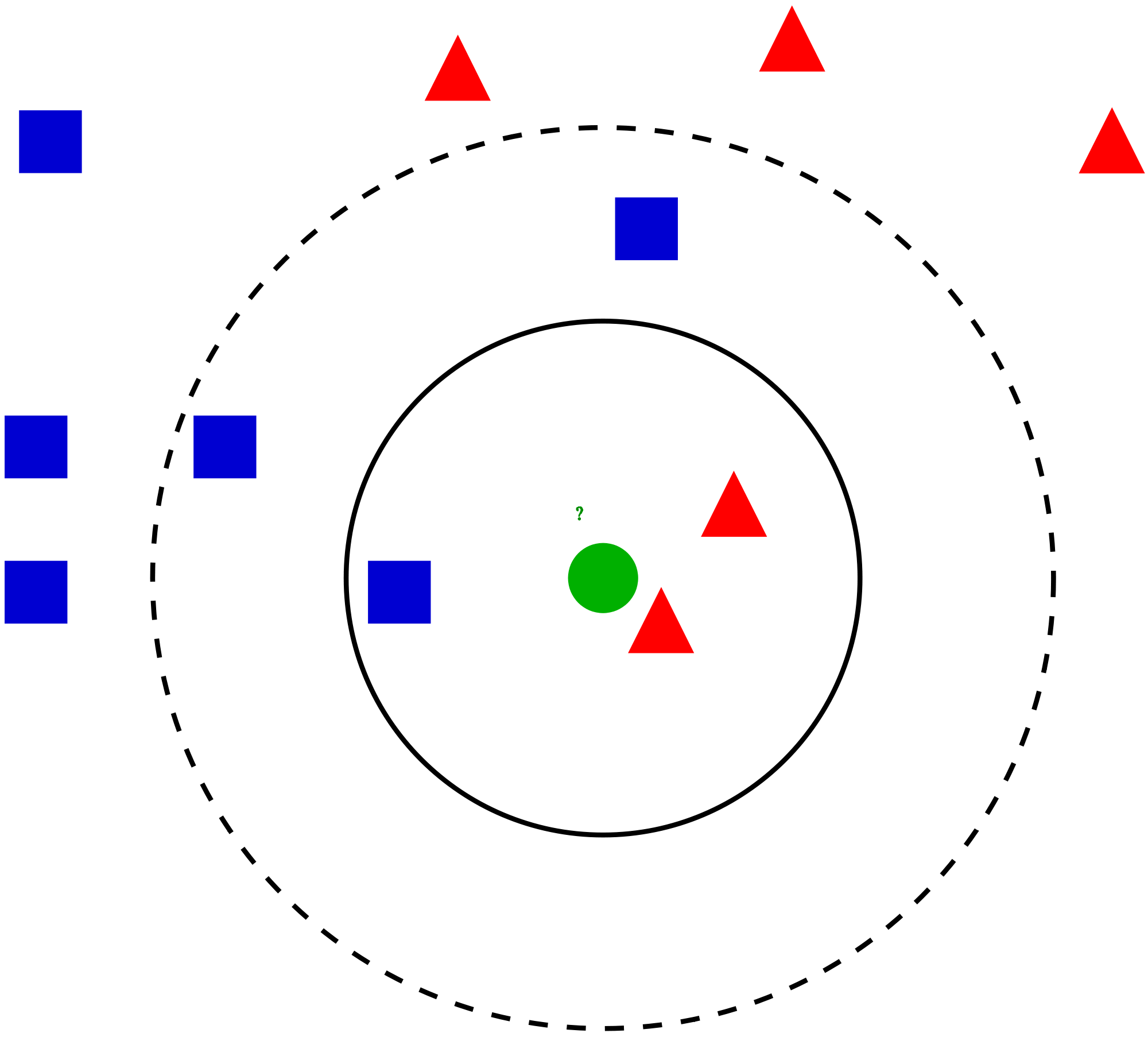In this article, we will talk about another widely used machine learning classification technique called K-nearest neighbors (KNN). Our focus will be primarily on how does the algorithm work and how does the input parameter affects the output/prediction.

Note: People whoo prefer to learn through videos can learn the same through our free course – K-Nearest Neighbors (KNN) Algorithm in Python and R. And if you are a complete beginner to Data Science and Machine Learning, check out our Certified BlackBelt program –

• When do we use KNN algorithm?
• How does the KNN algorithm work?
• How do we choose the factor K?
• Breaking it Down – Pseudo Code of KNN
• Implementation in Python from scratch
• Comparing our model with scikit-learn

## When do we use KNN algorithm?

KNN can be used for both classification and regression predictive problems. However, it is more widely used in classification problems in the industry. To evaluate any technique we generally look at 3 important aspects:

1. Ease to interpret output

2. Calculation time

3. Predictive Power

Let us take a few examples to  place KNN in the scale :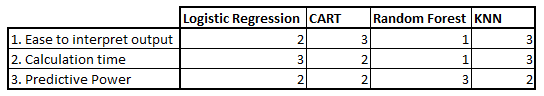KNN algorithm fairs across all parameters of considerations. It is commonly used for its easy of interpretation and low calculation time.

## How does the KNN algorithm work?

Let’s take a simple case to understand this algorithm. Following is a spread of red circles (RC) and green squares (GS) :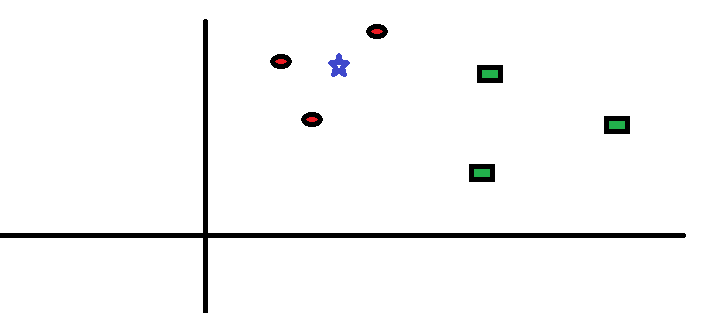You intend to find out the class of the blue star (BS). BS can either be RC or GS and nothing else. The “K” is KNN algorithm is the nearest neighbor we wish to take the vote from. Let’s say K = 3. Hence, we will now make a circle with BS as the center just as big as to enclose only three datapoints on the plane. Refer to the following diagram for more details: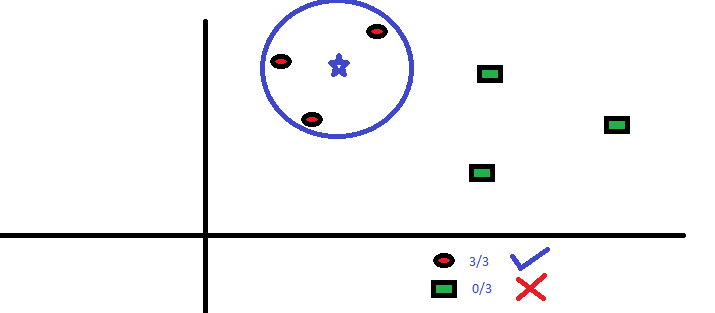The three closest points to BS is all RC. Hence, with a good confidence level, we can say that the BS should belong to the class RC. Here, the choice became very obvious as all three votes from the closest neighbor went to RC. The choice of the parameter K is very crucial in this algorithm. Next, we will understand what are the factors to be considered to conclude the best K.

## How do we choose the factor K?

First let us try to understand what exactly does K influence in the algorithm. If we see the last example, given that all the 6 training observation remain constant, with a given K value we can make boundaries of each class. These boundaries will segregate RC from GS. In the same way, let’s try to see the effect of value “K” on the class boundaries. The following are the different boundaries separating the two classes with different values of K.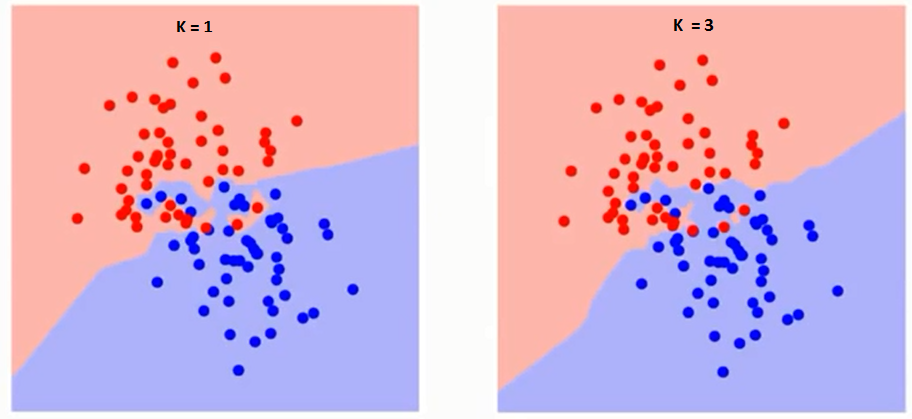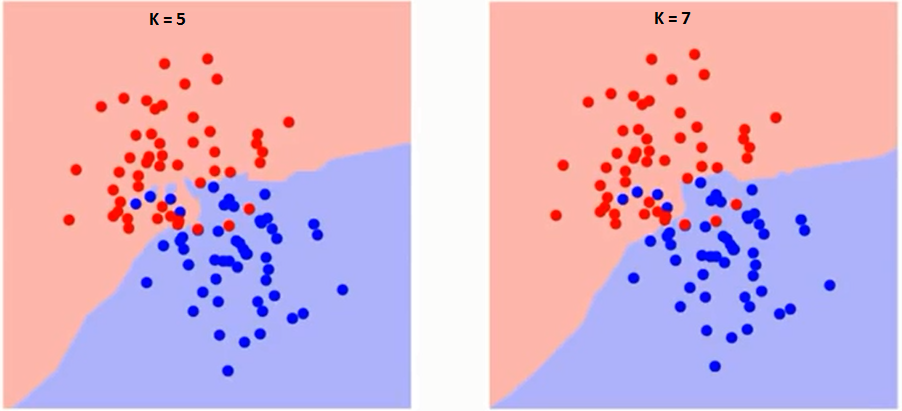If you watch carefully, you can see that the boundary becomes smoother with increasing value of K. With K increasing to infinity it finally becomes all blue or all red depending on the total majority.  The training error rate and the validation error rate are two parameters we need to access different K-value. Following is the curve for the training error rate with a varying value of K :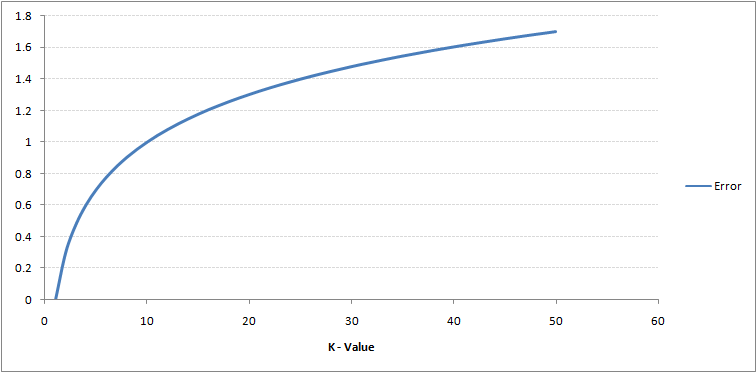As you can see, the error rate at K=1 is always zero for the training sample. This is because the closest point to any training data point is itself.Hence the prediction is always accurate with K=1. If validation error curve would have been similar, our choice of K would have been 1. Following is the validation error curve with varying value of K:This makes the story more clear. At K=1, we were overfitting the boundaries. Hence, error rate initially decreases and reaches a minima. After the minima point, it then increase with increasing K. To get the optimal value of K, you can segregate the training and validation from the initial dataset. Now plot the validation error curve to get the optimal value of K. This value of K should be used for all predictions.

The above content can be understood more intuitively using our free course – K-Nearest Neighbors (KNN) Algorithm in Python and R

## Breaking it Down – Pseudo Code of KNN

We can implement a KNN model by following the below steps:

2. Initialise the value of k
3. For getting the predicted class, iterate from 1 to total number of training data points
1. Calculate the distance between test data and each row of training data. Here we will use Euclidean distance as our distance metric since it’s the most popular method. The other metrics that can be used are Chebyshev, cosine, etc.
2. Sort the calculated distances in ascending order based on distance values
3. Get top k rows from the sorted array
4. Get the most frequent class of these rows
5. Return the predicted class

## Implementation in Python from scratch

We will be using the popular Iris dataset for building our KNN model. You can download it from here.

## Comparing our model with scikit-learn

```from sklearn.neighbors import KNeighborsClassifier
neigh = KNeighborsClassifier(n_neighbors=3)
neigh.fit(data.iloc[:,0:4], data['Name'])

# Predicted class
print(neigh.predict(test))

-> ['Iris-virginica']

# 3 nearest neighbors
print(neigh.kneighbors(test))
-> [[141 139 120]]

```

We can see that both the models predicted the same class (‘Iris-virginica’) and the same nearest neighbors ( [141 139 120] ). Hence we can conclude that our model runs as expected.

## Implementation of kNN in R

Step 1: Importing the data

Step 2: Checking the data and calculating the data summary

Output

```#Top observations present in the data
SepalLength SepalWidth PetalLength PetalWidth Name
1 5.1 3.5 1.4 0.2 Iris-setosa
2 4.9 3.0 1.4 0.2 Iris-setosa
3 4.7 3.2 1.3 0.2 Iris-setosa
4 4.6 3.1 1.5 0.2 Iris-setosa
5 5.0 3.6 1.4 0.2 Iris-setosa
6 5.4 3.9 1.7 0.4 Iris-setosa

#Check the dimensions of the data
 150 5

#Summarise the data
SepalLength SepalWidth PetalLength PetalWidth Name
Min. :4.300 Min. :2.000 Min. :1.000 Min. :0.100 Iris-setosa :50
1st Qu.:5.100 1st Qu.:2.800 1st Qu.:1.600 1st Qu.:0.300 Iris-versicolor:50
Median :5.800 Median :3.000 Median :4.350 Median :1.300 Iris-virginica :50
Mean :5.843 Mean :3.054 Mean :3.759 Mean :1.199
3rd Qu.:6.400 3rd Qu.:3.300 3rd Qu.:5.100 3rd Qu.:1.800
Max. :7.900 Max. :4.400 Max. :6.900 Max. :2.500

```

Step 3: Splitting the Data

Step 4: Calculating the Euclidean Distance

Step 5: Writing the function to predict kNN

Step 6: Calculating the label(Name) for K=1

Output

```For K=1
 "Iris-virginica"```

In the same way, you can compute for other values of K.

## Comparing our kNN predictor function with “Class” library

Output

```For K=1
 "Iris-virginica"```

We can see that both models predicted the same class (‘Iris-virginica’).

## End Notes

KNN algorithm is one of the simplest classification algorithm. Even with such simplicity, it can give highly competitive results. KNN algorithm can also be used for regression problems. The only difference from the discussed methodology will be using averages of nearest neighbors rather than voting from nearest neighbors. KNN can be coded in a single line on R. I am yet to explore how can we use KNN algorithm on SAS.

Did you find the article useful? Have you used any other machine learning tool recently? Do you plan to use KNN in any of your business problems? If yes, share with us how you plan to go about it.

### If you like what you just read & want to continue your analytics learning, subscribe to our emails, follow us on twitter or like our facebook page.

You can also read this article on our Mobile APP•Harshal says:

Useful article.
Can you share similar article for randomforest ?
What are limitations with data size for accuracy?

•Tavish Srivastava says:

Harshal,
We have already published many articles on random forest. Here is the link of the article on random forest on similar lines http://www.analyticsvidhya.com/blog/2014/06/introduction-random-forest-simplified/.

•saurabh says:

Good one please share the value of
Red circle and green square

•Tavish Srivastava says:

Saurabh,

The first graph is for illustrating purposes. You can create a random dataset to check the algorithm.

•DEBASHIS ROUT says:

I am currently doing part time MS in BI & Data Mining. I found this article is really helpful to understand in more detail and expecting to utilize in my upcoming project work. I need to know do you have any article on importance of Data quality in BI , Classification & Decision Tree.

•saraswathi says:

Hello
the article is very clear and precise. I would like some clarification on the single line
“To get the optimal value of K, you can segregate the training and validation from the initial dataset.”. Do you mean that we segregate those points on the border of the boundaries for validation and keep the remaining for training. This is not very clear to me. Can you please elaborate ?

Thanks.

•Tavish Srivastava says:

Saraswathi,

Here is what I meant : Take the entire population, and randomly split it into two. Now on the training sample, score each validation observation with different k-values. The error curve will give you the best value of k.

Hope it becomes clear now.

•saraswathi says:

I want to make sure I understand this correctly. Please confirm or correct.

You say, take the entire population and split into two – are these two divisions, one for training and one for validation ? ( I am assuming so).

So, now I use different values of K to cluster the training samples.

I try to see where the validation samples fall in these clusters.

I draw the error curve and choose the K with the smallest error ?

•Tavish says:

Saraswathi,
Let me make it even simpler. Say, you have 100 datapoints. Split this population into two samples of 70 and 30 observations. Use these 70 observation to predict for the other 30. Once you have the prediction for a particular value of k, check the misclassification with actual value. Repeat this exercise for different value of k. Hopefully, you will get a curve similar to that shown in the article. Now choose the k for which the misclassification is least.

Hope this makes it clear.

Tavish

•Harvey S says:

Nice tease: “KNN can be coded in a single line on R. ”

Can you give an example?

•Tavish Srivastava says:

We will cover this piece in our coming articles. Stay tuned.

•Felix says:

Hi, great post, thanks. I would like to add, that “low calculation time” is not true for the prediction phase with big, high dimensional datasets. But it’s still a good choice in many applications.

•Tavish says:

Felix,

You are probably right for cases where the distance between observations comparable in the large dataset. But in general population have natural clusters which makes the calculation faster. Let me know in case you disagree.

Tavish

•kabir singh says:

I am trying to figure out churn analysis, any suggestions where I am start looking?

BTW following this website for 6-8 months now, you guys are doing an amazing job

•Najma Naaz says:

That was very helpful. Thank you! Can you please share a concise article on neural nets and deep learning as well?

•Tiago says:

Thank you.

•Charles says:

•Aishwarya Singh says:
•thue xe du lich gia re says:

Quality articles or reviews is the secret to
invite the visitors to visit the website, that’s what this web site is providing.

•Emanuel Fakhar says:

The world of DS would be so boring and exaggerated without you guys. Anything I study, I get a better perspective from this site. And you are so generous and grounded compared to idiots here in UK. God bless.

•Stonehead Park says:

Excellent post, I appreciate your effort. 🙂

•Just81100 says:

KNN is fast to train but the prediction speed grows exponentially with the data set size and his complexity rather than Random forest…

•Soumya Shreya says:

It is a really nice and well explained article, I am a beginner in the field of data science and machine learning and I find these articles really helpful to learn and understand the algorithms. Thanks for publishing them.
Can you suggest me some datasets where I can experiment and apply KNN.

•Aishwarya Singh says:

Hi Soumya,

You can use the Cancer dataset to practice kNN.
Refer this link for the same.

•Krishna says:

How do we handle categorical features with kNN? Do we need to create dummies for them? Do you suggest any other distance method other than euclidean distance if we have more number of features? I feel we have to treat outliers as they may have impact on the distances, similarly missing values.. Please share your opinion.

•Aishwarya Singh says:

Hi,

Yes you can create dummies for categorical variables in kNN.
Apart from Euclidean distance, there are other methods that can be used to find the distance such as Manhattan or Minkowski.

•Aanish Singla says:

IMO limitation of KNN comes into play when dimensions increase because in higher dimensions, finding neighbors which are quite close to each other in all dimensions might be tough, hence so called neighbors might be really far apart from each other which defeats the purpose of the algorithm.

•Aishwarya Singh says:

Hi Aanish,

Thank you for sharing your thoughts.

•Amlesh Kanekar says:

I found it “inspiring”. Have spent last 4 months learning linear algebra, statistics, python. This learning list was culled from analyticsvidhya.com. Now just glimpsing through your article gives me the confidence to code knn from scratch. Thank you!

•Aishwarya Singh says:

Hi Amlesh,

•Amlesh Kanekar says:

I created my own dataset to experiment with KNN. When I plotted my data, the three targets/labels I have are extremely randomly distributed across the 2D plane … no clustering of the three colours is evident.
The Iris dataset shows a fairly high degree of clustering.
Should I continue with my dataset or there is the concept of “so-and-so distribution does not qualify for KNN”?
I can email a picture of my data plot if needed.

•Amlesh Kanekar says:

I figured this out. So it is fine if you do not respond.

•Max says:

That was very helpful. Thank you!
How to make the same visualization as in the pictures in section “How do we choose the factor K” ?

•Aishwarya Singh says:

Hi Max,

For this, you will have to use a for loop. For each value of k, calculate the validation error and store in a separate list. Then plot these validation error values against k values.

• […] learning technique and algorithm that can be used for both regression and classification tasks. K-Nearest Neighbors examines the labels of a chosen number of data points surrounding a target data point, in order to […]